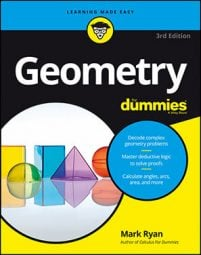##### Geometry For DummiesKnowing how to write two-column geometry proofs provides a solid basis for working with theorems. Practicing these strategies will help you write geometry proofs easily in no time:
• Make a game plan. Try to figure out how to get from the givens to the prove conclusion with a plain English, commonsense argument before you worry about how to write the formal, two-column proof.

• Make up numbers for segments and angles. During the game plan stage, it's sometimes helpful to make up arbitrary lengths for segments or measures for angles. Doing the math with those numbers (addition, subtraction, multiplication, or division) can help you understand how the proof works.

• Look for congruent triangles (and keep CPCTC in mind). In diagrams, try to find all pairs of congruent triangles. Proving one or more of these pairs of triangles congruent (with SSS, SAS, ASA, AAS, or HLR) will likely be an important part of the proof. Then you'll almost certainly use CPCTC on the line right after you prove triangles congruent.

• Try to find isosceles triangles. Glance at the proof diagram and look for all isosceles triangles. If you find any, you'll very likely use the if-sides-then-angles or the if-angles-then-sides theorem somewhere in the proof.

• Look for parallel lines. Look for parallel lines in the proof's diagram or in the givens. If you find any, you'll probably use one or more of the parallel-line theorems.

• Look for radii and draw more radii. Notice each and every radius of a circle and mark all radii congruent. Draw new radii to important points on the circle, but don't draw a radius that goes to a point on the circle where nothing else is happening.

• Use all the givens. Geometry book authors don't put irrelevant givens in proofs, so ask yourself why the author provided each given. Try putting each given down in the statement column and writing another statement that follows from that given, even if you don't know how it'll help you.

For each reason, check that

• All the ideas in the if clause appear in the statement column somewhere above the line you're checking.

• The single idea in the then clause also appears in the statement column on the same line.

You can also use this strategy to figure out what reason to use in the first place.

• Work backward. If you get stuck, jump to the end of the proof and work back toward the beginning. After looking at the prove conclusion, make a guess about the reason for that conclusion. Then use your if-then logic to figure out the second-to-last statement (and so on).

• Think like a computer. In a two-column proof, every single step in the chain of logic must be expressed, even if it's the most obvious thing in the world. Doing a proof is like communicating with a computer: The computer won't understand you unless every little thing is precisely spelled out.

• Do something. Before you give up on a proof, put whatever you understand down on paper. It's quite remarkable how often putting something on paper triggers another idea, then another, and then another. Before you know it, you've finished the proof.